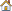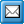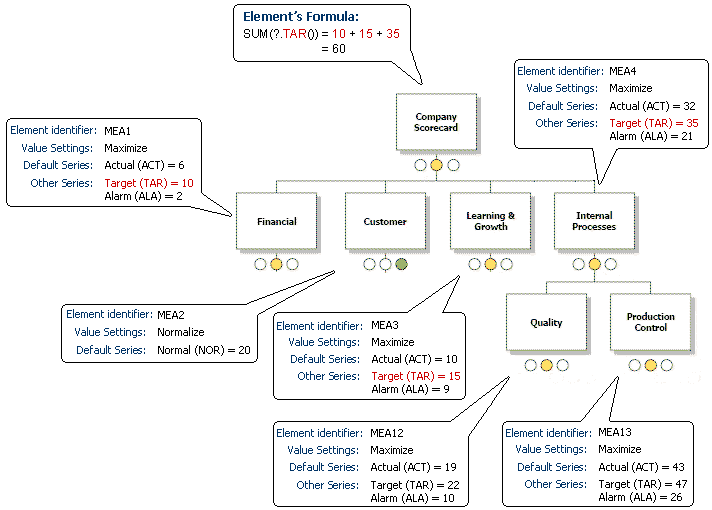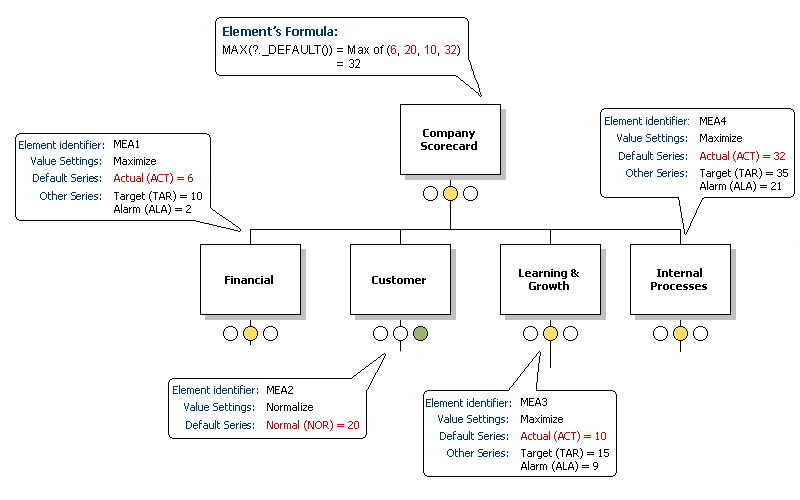﻿ How Formulas Work in Practice
QPR Knowledge Base 2017.1

How Formulas Work in PracticeMetrics User's Guide > Using QPR Metrics > Formulas:

How Formulas Work in PracticeHow Formulas Work in PracticeIn the following example, the top element Company Scorecard has the formula SUM(?.TAR()). The "?.TAR()" part of the formula takes the TAR (Target) value for the direct sub-elements of the element Company Scorecard. Since only three of its four sub-elements has the series Target (element identifier TAR), when the element Company Scorecard's value for period Q4 2002 is calculated, it only gets three values (10, 15, and 35), which sum up to 60.

Note: If you wish to add elements to a formula in the manner of SUM(?.TAR())+2, for example, the formula will calculate the sum of the target values first and then add the 2 to the final sum. It will not add the 2 to all the Target values before summing them up.

You need to pay attention to the positioning of operands, as the functionality of the formula may change with another positioning. For example, if the previous example was written like SUM(?.TAR()+2), it would sum up all the Target series values and add the 2 to the last Target value. In this case with SUM, the outcome would be the same, but if the formula was AVERAGE instead of SUM, it would yield different results depending on how the operands are positioned.

Period: Q4 2002In the following example, the top element Company Scorecard has the formula MAX(*._DEFAULT()). The "?._DEFAULT()" part of the formula takes the values of the default series from all the direct sub-elements of the element Company Scorecard. When the element Company Scorecard's value for period Q4 2002 is calculated, it gets its values from the default series of its direct sub-elements: Financial (Actual = 6), Customer (Normal = 6), Learning & Improvement (Actual=10), Processes (Actual = 32). Therefore Company Scorecard's formula calculates the maximum of these four values to be 32.

Note: Since elements can use different value settings, they can have different default series. However, with the "_DEFAULT" parameter, the name of the series is not required, the formula simply takes the default series for the element, regardless of which series it is.

Period: Q4 2002For examples of various formulas, see Formula Examples.

For details on how to define a formula for an element, see Formula Window.

For a list of the build-in functions, see Functions.

For information about the arithmetic and logical operators, see Operators.

For information about expressions, see Expressions.

For information about constants, see Constants.

For information about wildcards, see Wildcards.

For information about calculating the values of the functions, see Calculation.

For information about error conditions with formulas, see Error Handling.